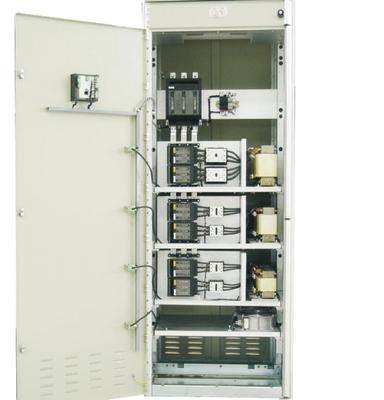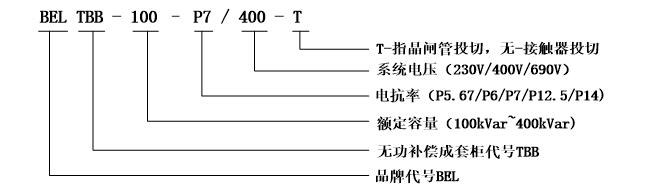# 低压无功补偿成套装置u   每个补偿支路包括：熔断器、电容器、电抗器、接触器或可控硅投切开关；

u   功率因数自动补偿控制器，液晶显示屏配置手动和自动模式；

u   前门装有控制器的标准配电柜，导线端子及风扇；

u   不同的安装方式：模块式安装，组件式安装；

u   不同的补偿方案：三相共补，分相补偿，混合补偿等；

u   目前市场可选柜型GGDGCKGCSMNS等；功率（kVar） 型号 补偿回路容量 W*D*H（mm） 100 BELTBB-100-PX/400 25+25+25+25 800*600*2200 150 BELTBB-150-PX/400 25+25+50+50 800*600*2200 200 BELTBB-200-PX/400 25+25+50+50+50 800*600*2200 250 BELTBB-250-PX/400 25+25+50+50+50+50 800*800*2200 300 BELTBB-300-PX/400 25+25+50+50+50+50+50 1000*1000*2200 350 BELTBB-350-PX/400 25+25+50+50+50+50+50+50 1000*1000*2200 400 BELTBB-400-PX/400 50+50+50+50+50+50+50+50 1000*1000*2200

 功率（kVar） 型号 补偿回路容量 W*D*H（mm） 100 BELTBB-100-PX/400-T 25+25+25+25 800*600*2200 150 BELTBB-150-PX/400-T 25+25+50+50 800*600*2200 200 BELTBB-200-PX/400-T 25+25+50+50+50 800*600*2200 250 BELTBB-250-PX/400-T 25+25+50+50+50+50 800*800*2200 300 BELTBB-300-PX/400-T 25+25+50+50+50+50+50 1000*800*2200 350 BELTBB-350-PX/400-T 25+25+50+50+50+50+50+50 1000*1000*2200 400 BELTBB-400-PX/400-T 50+50+50+50+50+50+50+50 1000*1000*2200

 功率（kVar） 型号 补偿回路容量 W*D*H（mm） 100 BELTBB-300-P7/690 25+25+25+25 800*800*2200 150 BELTBB-300-P7/690 25+25+50+50 800*800*2200 200 BELTBB-300-P7/690 25+25+50+50+50 800*800*2200 250 BELTBB-300-P7/690 25+25+50+50+50+50 1000*1000*2200 300 BELTBB-300-P7/690 25+25+50+50+50+50+50 1000*1000*2200